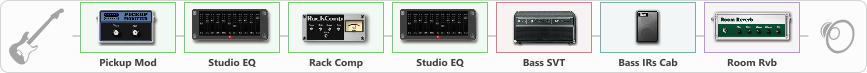# bass simulator

Discussion in 'ToneLib-GFX presets' started by badtz_maru, Dec 9, 2022.

1. bass simulator

Preset name: bass sim

Use the low strings (E A D), if U dont have a bassguitar

Effects chain:Effect: "Pickup Mod" (Dynamics / Filter), active - "yes"
{
"Mode" = Humbacker->Single
"Tone" = 50
}

Effect: "Studio EQ" (Dynamics / Filter), active - "yes"
{
"31 Hz" = -15
"62 Hz" = 6
"125 Hz" = 8
"250 Hz" = -15
"500 Hz" = -15
"1 kHz" = -15
"2 kHz" = -15
"4 kHz" = -15
"8 kHz" = -15
"16 kHz" = -15
"Level (dB)" = 0
}

Effect: "Rack Comp" (Dynamics / Filter), active - "yes"
{
"Threshold (dB)" = -17
"Ratio" = 10
"Attack" = Fast
"Release (ms)" = 232
"Knee" = 38
"Level (dB)" = 0
}

Effect: "Studio EQ" (Dynamics / Filter), active - "yes"
{
"31 Hz" = -15
"62 Hz" = 0
"125 Hz" = 0
"250 Hz" = -15
"500 Hz" = -15
"1 kHz" = -15
"2 kHz" = -15
"4 kHz" = -15
"8 kHz" = -15
"16 kHz" = -15
"Level (dB)" = 0
}

Effect: "Bass SVT" (Amp simulators), active - "yes"
{
"Bass Ctrl" = UltraLo
"Midrange" = 200 Hz
"Ultra Hi" = Off
"Bass" = 0
"Middle" = 0
"Treble" = 0
"Volume" = 37
"Level (dB)" = 0
}

Effect: "Bass IRs Cab" (Cabinets), active - "yes"
{
"Model" = Ampeg SVT 810e (8x10")
"Mic Position" = Middle
"Mic Distance" = Far
"Low Cut (Hz)" = 0
"Hi Cut (kHz)" = 20.0
"Mix" = 100
"Level (dB)" = 0
}

Effect: "Room Rvb" (Reverberation), active - "yes"
{
"Time" = 4.5
"PreDelay" = 0
"LoDamp" = 46
"HiDamp" = 25
"Mix" = 59
}

Note: You will need to download and install the ToneLib-GFX software to use the preset.

File size:
782 bytes
Views:
3,320